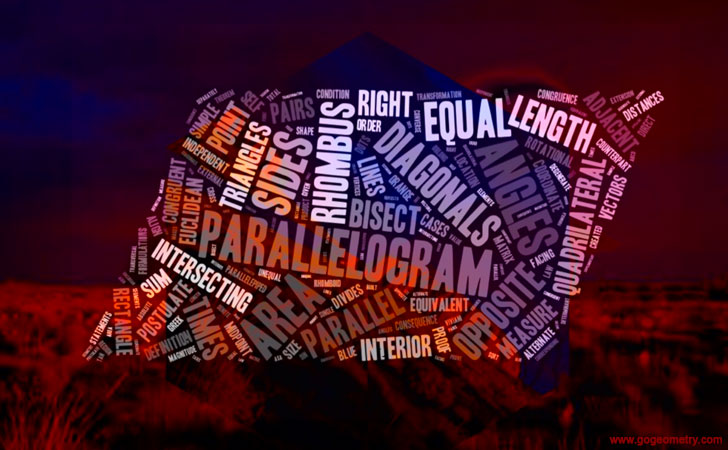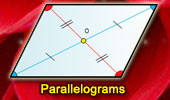# Plane Geometry: Parallelogram Theorems and Problems. Page 5Parallelogram, Theorems and Problems - 5Triangle and Squares. 21 theorems. Visual illustrations. Eyeball Theorem: Animated Angle to Geometry Study. Varignon and Wittenbauer parallelograms. Quadrilateral: midpoints and trisection points of the edges. Van Aubel's Theorem. Quadrilateral with Squares. Proof with animation. Sangaku Problem. The incenters of four triangles in a cyclic quadrilateral form a rectangle. Proposed Problem 35. Incenters and Inradii in Cyclic Quadrilateral. Go to page: Previous | 1 | 2 | 3 | 4 | 5 | Next

 Home | Search | Geometry | Quadrilateral | Email | By Antonio Gutierrez